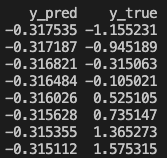# Pytorch regression model cannot learn

Hi,
for a while I’ve been using for classification task a model, that first prepares features from some time series(LSTM) and then has a few fully connected layers to get actual predictions. Now I took the same architecture to predict regression task and the model cannot learn anything. I simplified task to input series of length 1, and basically it need to learn x=y, but cannot.
I checked that gradients are basically zeros and it predicts just using biases (so all predictions are almost the same).

I checked some solutions I found online: standarization of inputs and outputs, took out dropout, BatchNorm, experimented with lr, weight_decay, other losses, different weights initializations, other architectures, removing rnn with no success. Maybe sb had such issue?
Inputs:Predictions:Bellow you can see the simplified code:

``````import random
import pandas as pd
import torch
import torch.nn as nn
import pytorch_lightning as pl
from pytorch_lightning import Trainer
from pytorch_lightning.loggers import WandbLogger

pl.seed_everything(1234, workers=True)

# Prepare mock dataset
df = pd.DataFrame(
{
"id": [f"x_{i}" for i in range(0, 16)],
"prediction_date": [f"{y}-01-01" for y in [2018, 2019, 2020, 2021] * 4],
"x": [x + random.uniform(0, 0.2) for x in range(0, 16)],
"y": [x for x in range(0, 16)],
}
)
df["prediction_date"] = pd.to_datetime(df["prediction_date"])

# Standardize inputs and outputs
df["x"] = (df["x"] - df["x"].mean()) / df["x"].std()
df["y"] = (df["y"] - df["y"].mean()) / df["y"].std()

# Train-test split
X_train = df[df["prediction_date"] < "2020-01-01"]["x"]
y_train = df[df["prediction_date"] < "2020-01-01"]["y"]
X_val = df[df["prediction_date"] >= "2020-01-01"]["x"]
y_val = df[df["prediction_date"] >= "2020-01-01"]["y"]

print(df)

class Regression(pl.LightningModule):
def __init__(self):
super(Regression, self).__init__()

self.rnn = nn.LSTM(input_size=1, hidden_size=300, num_layers=2)
self.fc1 = nn.Linear(300, 10)
self.activation = nn.ReLU()
self.fc2 = nn.Linear(10, 1)

self.mse_loss = nn.MSELoss(reduction="mean")

train_dataset = TensorDataset(
torch.tensor(X_train.values).float(), torch.tensor(y_train.values).float()
)

validation_dataset = TensorDataset(
torch.tensor(X_val.values).float(), torch.tensor(y_val.values).float()
)
dataset=validation_dataset, batch_size=8, shuffle=False
)

def configure_optimizers(self):

def forward(self, x):
x = self.rnn(x.unsqueeze(1).unsqueeze(1))
x = self.fc1(x)
x = self.activation(x)
x = self.fc2(x)
return x

def training_step(self, batch, batch_idx):
x, y = batch
y_hat = self.forward(x)
loss = self.mse_loss(y_hat, y)
# self.log_data("train_loss", loss)
return {"loss": loss}

def validation_step(self, batch, batch_idx):
x, y = batch
y_hat = self.forward(x)
loss = self.mse_loss(y_hat, y)
# self.log_data("val_loss", loss)
return {"val_loss": loss}

def predict_step(self, batch, batch_idx, dataloader_idx: int = None):
x, y = batch
y_hat = self.forward(x)
return y_hat

def log_data(self, name: str, data: torch.Tensor):
self.log(
name,
data,
on_epoch=True,
on_step=False,
logger=True,
prog_bar=False,
)

wandb_logger = WandbLogger(
**{"name": "test",}
)

model = Regression()
tr = Trainer(max_epochs=200)  # , logger=wandb_logger, track_grad_norm=2)
tr.fit(model)

out = pd.DataFrame({"y_pred": preds.squeeze().cpu().numpy(), "y_true": y_val.values})
print(f"MAE: {round((out['y_pred'] - out['y_true']).abs().mean(), 2)}")
print()
print(out)
``````

Based on your code snippet it seems you are returning samples from the `DataLoader` in the shape `[batch_size=8]` and are thus unsqueezing the input before passing it to the `nn.LSTM`.
In this case, I guess the model output would have the shape `[batch_size, 1]` while the target should still have the shape `[batch_size]`, which would then apply an unwanted broadcasting and will raise a warning which you might have ignored:

``````x, y = next(iter(train_loader))
mse_loss = nn.MSELoss(reduction="mean")
output = torch.randn(8, 1)
loss = mse_loss(output, y)
# > UserWarning: Using a target size (torch.Size()) that is different to the input size (torch.Size([8, 1])). This will likely lead to incorrect results due to broadcasting. Please ensure they have the same size.
``````

@ptrblck thanks, actually this problem was only in the snippet I prepared. In the actual model it was correct, nevertheless thanks a LOT because pointing this out helped me realize that there was still sth different between snippet that was already working and the actual model.
Maybe it might help sb in the future, the problem was caused by some legacy logging of features: `num_feat[num_feat > 0] = num_feat[num_feat > 0].log()` - avoid such things